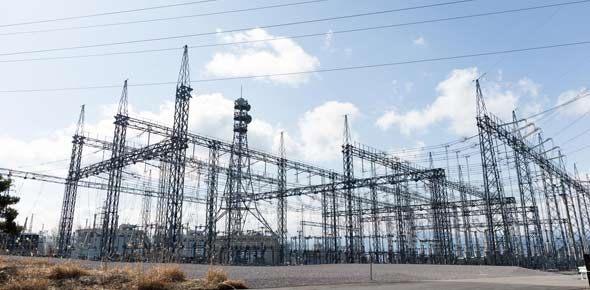Chapter 33. Electric Fields And Potential

8 Questions | Total Attempts: 338SettingsSame as the other quiz.

• 1.
The force field that surrounds a mass is a gravitational field.
• A.

True

• B.

False

• 2.
The SI unit of measurement for electric potential is the joule.
• A.

True

• B.

False

• 3.
A kind of aura that extends through space is an/a  ____________.
• A.

Force field

• B.

Electric field

• C.

Gravitational field

• 4.
Since an electric field has both magnitude and direction, it is a _________.
• A.

Scalar quantity

• B.

Translational quantity

• C.

Vector quantity

• 5.
Electrical energy can be stored in a common device called a __________.
• A.

Capacitor

• B.

Transistor

• C.

Semiconductor

• 6.
There is no way to shield gravity, because gravity only _______.
• A.

Repels

• B.

Suspends

• C.

Attracts

• 7.
Why are the occupants safe inside a car struck by lightning?
• 8.
What is the difference between electrical potential energy and electrical potential?
Related TopicsBack to top